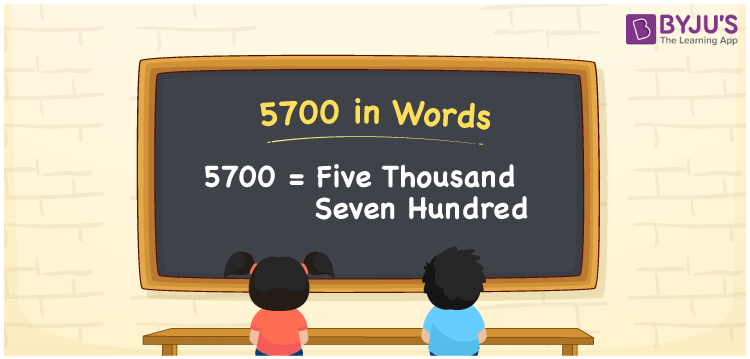# 5700 in words

5700 in words is written as Five Thousand Seven Hundred. 5700 represents the count or value. The article on Counting Numbers can give you an idea about count or counting. The number 5700 is used in expressions that relate to money, distance, length and others. Let us consider an example for 5700. “An artist used Five Thousand Seven Hundred Dominos for an art”. Another example ”My hard disk has a collection of Five Thousand Seven Hundred movies and shows.”

 5700 in words Five Thousand Seven Hundred Five Thousand Seven Hundred in Numbers 5700

## 5700 in English Words## How to Write 5700 in Words?

We can convert 5700 to words using a place value chart. This can be done as follows. The number 5700 has 4 digits, so let’s make a chart that shows the place value up to 4 digits.

 Thousands Hundreds Tens Ones 5 7 0 0

Thus, we can write the expanded form as:

5 × Thousand + 7 × Hundred + 0 × Ten + 0 × One

= 5 × 1000 + 7 × 100 + 0 × 10 + 0 × 1

= 5700

= Five Thousand Seven Hundred.

5700 is the natural number that is succeeded by 5699 and preceded by 5701.

5700 in words –FIve Thousand Seven Hundred.

Is 5700 an odd number? – No.

Is 5700 an even number? – Yes.

Is 5700 a perfect square number? – No.

Is 5700 a perfect cube number? – No.

Is 5700 a prime number? – No.

Is 5700 a composite number? – Yes.

## Solved Example

1. Write the number 5700 in expanded form

Solution: 5 × 1000 + 7 × 100 + 0 × 10 + 0 × 1.

We can write 5700 = 5000 + 700 + 0 + 0

= 5 × 1000 + 7 × 100 + 0 × 10 + 0 × 1.

## Frequently Asked Questions on 5700 in words

Q1

### How to write 5700 in words?

5700 in words is written as Five Thousand Seven Hundred.
Q2

### State whether True or False. 5700 is divisible by 3?

True. 5700 is divisible by 3.
Q3

### Is 5700 divisible by 10?

Yes. 5700 is divisible by 10.